Open in App
Not now

# Represent √3 on the number line

• Last Updated : 29 Sep, 2021

A number line is a line that is used to represent numbers at specific intervals, which are used for simple numerical operations. Natural numbers, whole numbers, integers, rational and irrational numbers are types of numbers. These are all part of the Real numbers or the Real number line. All these numbers could be represented visually using a number line? The positive integers after zero, that are used for counting are known as natural numbers. Remember that they are denoted by the symbol ‘N‘. Now add zero to this collection. you now have the collection of whole numbers which is denoted by the symbol ‘W’. Now the collection of the vast stretch of negative integers before zero and the whole numbers is the collection of all integers, and it is denoted by the symbol ‘Z’. You might wonder, why Z? Z comes from the German word ‘Zahlen”, which means “numbers”. Are there any numbers still left on the number line? Yes, Of course, there are many different numbers like 1/3, -6352/371, 25/6. If all these numbers are included with the collection of integers, it will now be the collection of Rational numbers. Recall that rational numbers are denoted by ‘Q’. Why Q? Rational comes from the word ‘ratio’ and Q comes from the word ‘quotient’. One might wonder, where do irrational numbers fall in this number line? The numbers which are not rationales were classified under irrational numbers. The Pythagoreans, the followers of the famous mathematician and Greek philosopher Pythagoras, were the first to find irrational numbers.

### Types of Numbers

There are different types of numbers. As mentioned above, Integers, natural numbers, whole numbers, rational numbers, irrational numbers, etc. Let’s see some points about these specific types,

Natural numbers

• These are the numbers that are used for counting.
• 1, 2, 3, 4… so on and so forth.
• They are denoted by the symbol ‘N’.

Whole Numbers

• Whole numbers are a set of natural numbers including ZERO (0).
• For eg. 9, 35, 100, 0 etc.
• They are denoted by the symbol ‘W’.

Integers:

• Integers are a set of positive and negative numbers including zero. They do not have a decimal or a fractional component.
• For eg. 24, -18, 0, -1, 8 etc.
• They are denoted by the symbol ‘Z’.

Rational Numbers

• A set of numbers that can be represented in the form of p/q, where p and q are integers and q is not equal to 0 is called Rational numbers.
• For eg. 25/5. 17/8, -3/8.
• They are denoted by the symbol ‘Q’.

Irrational numbers

• A set of numbers that cannot be represented in the form p/q, where p and q are integers and q is not equal to zero is called Irrational numbers.
• For eg. Pi (π), The golden ratio (1.618033….), √2, √3, etc.
• They are denoted by the symbol ‘P’.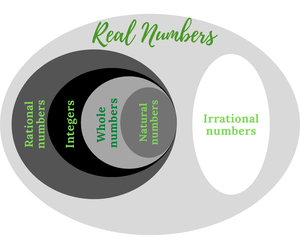Real numbers pie chart

### Difference between Rational and Irrational numbers

The first and foremost thing to remember is that the decimal places of Rational numbers are either terminating or recurring. Whereas the decimal places of Irrational numbers are non-terminating and non-recurring.

### Represent √3 on the number line

Method 1

Representation of √2 on the number line

Step 1: Draw a line segment of 1cm anywhere on a number line.

Step 2: Mark the line segment A and B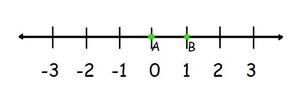Step 3: Draw a line segment of 1cm perpendicular to AB, and mark the point C.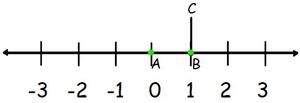Step 4: Draw a line connecting A and C.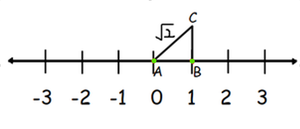Step 5: AC is √(12 + 12) = √2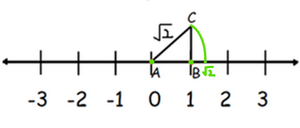Representation of √3 on the number line

Step 6: Draw a line segment of 1cm perpendicular to AC. Mark the point D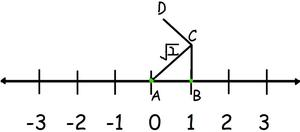Step 7: Draw line connecting A and D.

Step 8: AD is √ {(√2)2 + (1)2}= √3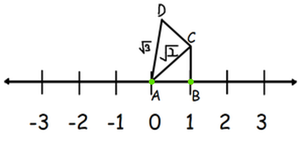Step 9: Take a compass and take the radius to the length of AD.

Step 10: Extend the arc and mark where it touches the number line as E

Step 11: AE is √3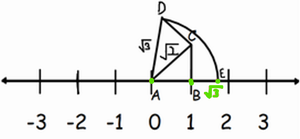Similarly, locate √n for any positive integer n, after √n-1 has been located. Continue doing this for √4, √5, √6, etc. Square root spiral is obtained.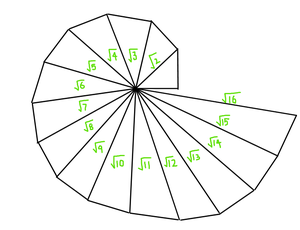Square root spiral

Method 2

Representation of √2 on the number line.

Method 1 is tedious and time-consuming. It is easy to use for smaller numbers. Therefore there is another easy way to represent irrational numbers on the number line which is less time-consuming and can also be used for larger numbers.

Step 1: Draw a line segment of n cm. In this case, it’s 2 cm, and mark them A and B.

Step 2: Extend B to C to form a line segment of 1cm.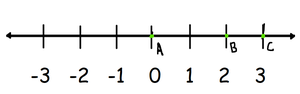Step 3: Take a compass, and mark an arch, placing one end of the compass in A. With the same radius, draw another arch placing one end of the compass in B.

Step 4: Mark the point of intersection between the arches as D.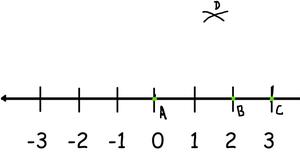Step 5: Draw a perpendicular line joining D and AC. Mark this point as E.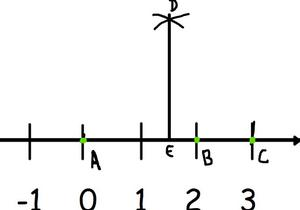Step 6: Take AE as radius, and draw a semicircle.

Step 7: Draw a perpendicular line joining B and the semicircle, and mark the point F.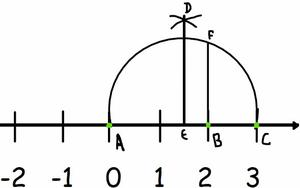Step 8: BF is √2

Step 9: Take radius AF on the compass on extending it till the number line, and mark the point G.

Step 10: BG is √2 = 1.41421…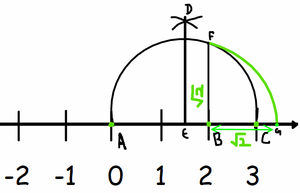Using the same steps you can represent √n on the number line, by taking AB as n cm.

Representation of √3 on the number line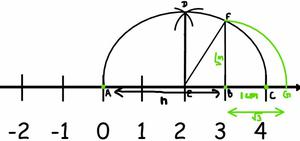AB = n units, BC = 1 unit.

In this case, n = 3.

BF is = √n

We can prove this by using the Pythagoras Theorem. Note that △EBF is a right-angled triangle and the radius of the circle is (n + 1)/2 units. Therefore, EC = EF = EA = (n + 1)/2 units.

OB = n – (n + 1/2) = (n – 1)/2.

Applying the Pythagoras theorem,

(BF)2 – (EF)2 – (EB)2 = {(n + 1)/2}2 = (4n)/4 = n.

This proves that BF = √n.

### Sample Problems

Question 1: Representation of √4 on the number line.

Solution: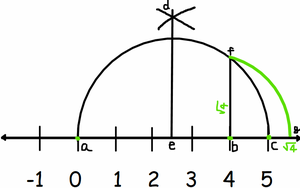Question 2: Representation of √5 on the number line.

Solution: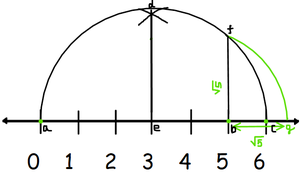This construction gives us a visual, and geometric way of showing that √n exists for all real number n>0.

My Personal Notes arrow_drop_up